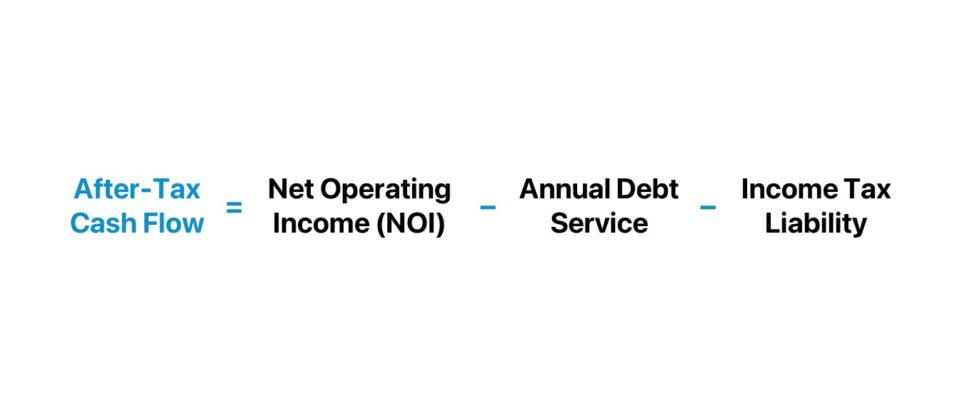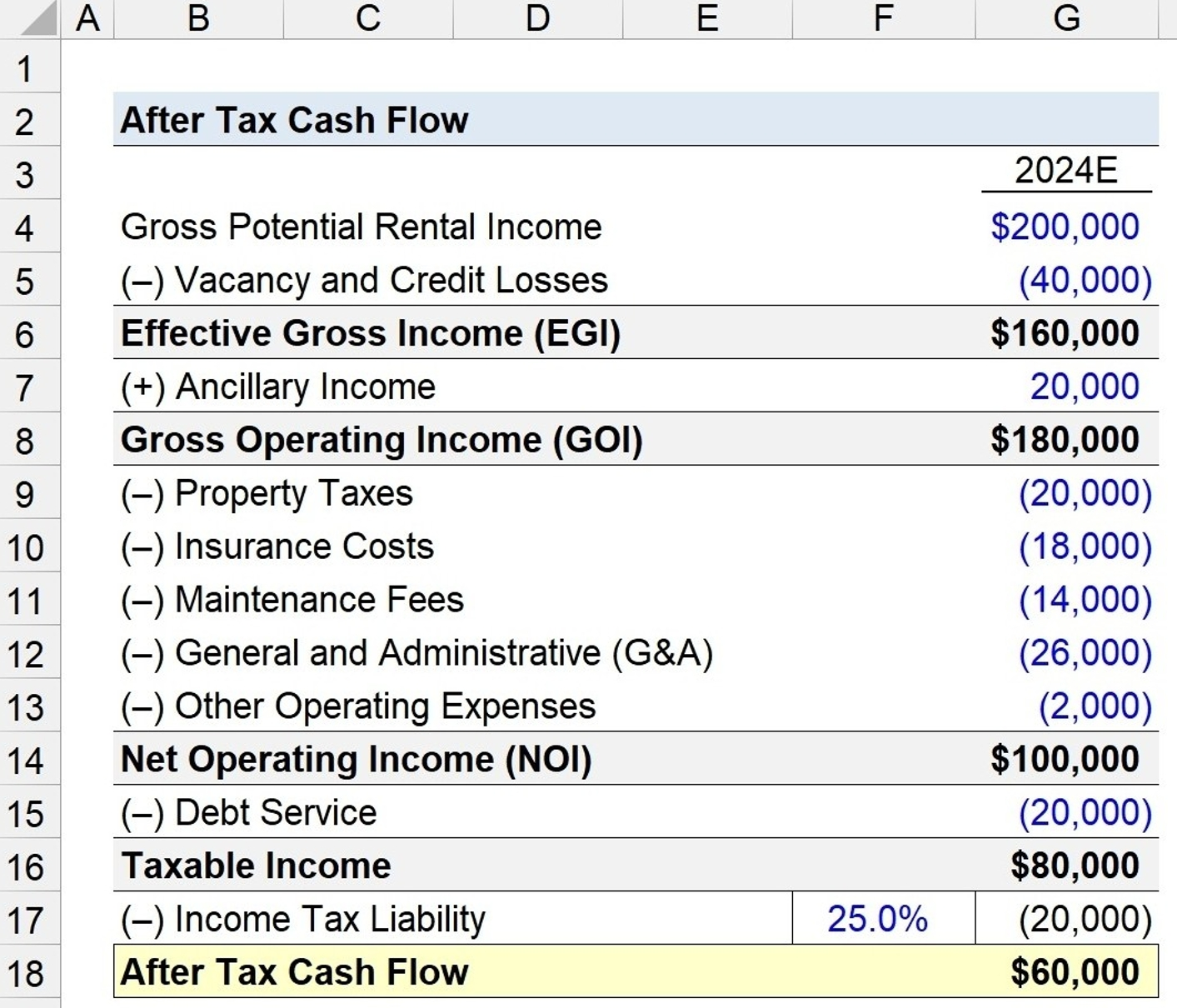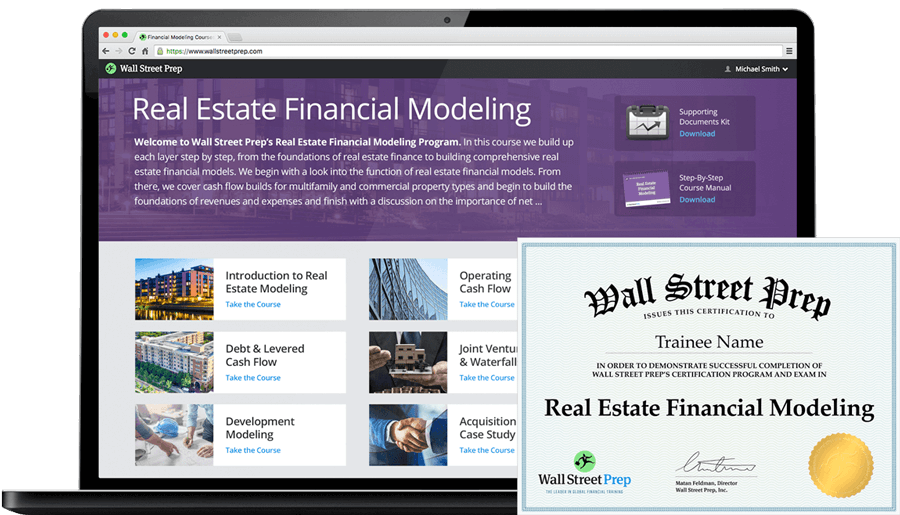Welcome to Wall Street Prep! Use code at checkout for 15% off.# After Tax Cash Flow (ATCF)

Guide to Understanding After-Tax Cash Flow in Real Estate## How to Calculate After Tax Cash Flow?

The after-tax cash flow is the income left over after taxes have been paid.

In commercial real estate (CRE) investing, the after-tax cash flow is a metric that measures the remaining income once a property’s operating expenses, debt service obligations, and income taxes (IRS) have been fulfilled.

The after-tax cash flow of a property investment can be categorized as an indicator of profitability, since the metric measures the discretionary income remaining after the annual income tax liability has been satisfied.

Therefore, the after-tax cash flow is determined by calculating the taxable income of a real estate property, and then subtracting the annual income tax provision.

The residual cash flow belongs to the real estate investor, since the obligations owed to third parties, such as the taxes owed to the Internal Revenue Service (IRS), have been met.

While the jurisdiction of the property and surrounding details can cause the tax obligation to shift, most taxpayers are permitted to apply certain deductions to their taxable income, most often mortgage interest payments and depreciation.

The process of calculating the after-tax cash flow metric is a three-step process:

1. Compute Taxable Income → The first step is to calculate the property’s taxable income in the current period. The taxable income equals the property’s net operating income (NOI) minus interest expense owed on financial borrowings, depreciation allowance, and amortized loan costs.
2. Determine Tax Liability → Once the appropriate adjustments have been applied, the next step is to multiply the taxable income by the marginal tax rate, resulting in the tax liability for the given period.
3. Calculate After Tax Cash Flow → In the final step, the tax liability is subtracted from the taxable income of the property to arrive at the after-tax cash flow.## After Tax Cash Flow Formula

The formula to calculate the after tax cash flow is as follows.

After-Tax Cash Flow = Net Operating Income (NOI)  Annual Debt Service Income Taxes

Where:

• Net Operating Income (NOI) = (Rental Income + Ancillary Income) – Direct Operating Expenses
• Annual Debt Service = Σ Current Principal Amortization + Interest Expense
• Income Tax = Marginal Tax Rate (%) × (NOI – Annual Debt Service)

## After Tax Cash Flow Calculator

We’ll now move to a modeling exercise, which you can access by filling out the form below.#### Get the Excel Template!Submitting...

## Real Estate After Tax Cash Flow Calculation Example

Suppose we’re presented with the following pro forma operating performance of a real estate rental property to calculate the after-tax cash flow.

Pro Forma Operating Performance 2024E
Gross Potential Rental Income \$200,000
(–) Vacancy and Credit Losses (\$40,000)
Effective Gross Income (EGI) \$160,000
(+) Ancillary Income \$20,000
Gross Operating Income (GOI) \$180,000
(–) Property Taxes (\$20,000)
(–) Insurance Costs (\$18,000)
(–) Maintenance Fees (\$14,000)
(–) General and Administrative (G&A) (\$26,000)
(–) Other Operating Expenses (\$2,000)
Net Operating Income (NOI) \$100,000

In 2024, the property is forecast to generate \$160k in effective gross income (EGI), \$180k in gross operating income, and \$100k in net operating income (NOI).

The next step is to deduct non-operating items, namely the annual debt service, which we’ll assume is \$20k.

• Annual Debt Service = \$20,000

The taxable income, or before-tax cash flow (BTCF), of the real estate property is \$80k.

• Taxable Income = \$100,000 – \$20,000 = \$80,000

In the closing steps of our exercise, we’ll subtract the income tax liability from the property’s taxable income, assumed to be 25.0%, inclusive of all relevant non-operating taxes. The income tax liability is \$20k, after multiplying the taxable income by the marginal tax rate.

• Marginal Tax Rate (%) = 25.0%
• Income Tax Liability = 25.0% × \$80,000 = \$20,000

In conclusion, the pro forma after-tax cash flow of our hypothetical property is \$60k, upon subtracting its estimated income tax liability from its projected taxable income.

• After Tax Cash Flow = \$80,000 – \$20,000 = \$60,00020+ Hours of Online Video Training

### Master Real Estate Financial Modeling

This program breaks down everything you need to build and interpret real estate finance models. Used at the world's leading real estate private equity firms and academic institutions.

Inline Feedbacks